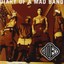MIT 6.00 Intro Computer Science (OCW)OpenStudy (anonymous):

solutions to problem set 1.... please just post solution... been trying for a few days nowOpenStudy (anonymous):

both problemsOpenStudy (anonymous):

Here is what i finally managed to get a hold of for part 1: def primes(n): """ returns a list of prime numbers from 2 to n """ if n < 2: return [] if n == 2: return  # create a list of odd numbers from 3 to n nums = list(range(3, n+1, 2)) nums_len = (n // 2) - 1 + (n % 2) idx = 0 idx_sqrtn = (int(n**0.5) - 3) // 2 while idx <= idx_sqrtn: nums_idx = (idx << 1) + 3 for j in range(idx*(nums_idx+3)+3, nums_len, nums_idx): # if not a prime replace with zero nums[j] = 0 idx += 1 while idx <= idx_sqrtn: if nums[idx] != 0: break idx += 1 # remove all the zero entries return  + [x for x in nums if x != 0] print('-' * 50) # print 50 dashes, cosmetic num = 7919 primeList = primes(num) print("List of prime numbers from 2 to %d:" % num) print(primeList)

Latest QuestionsKyledaGreat: Your backpacker's guide contains a grid map of Paris, with each unit on the grid representing 0.
2 minutes ago 3 Replies 0 MedalsTETSXPREME: help
16 minutes ago 1 Reply 0 MedalsNoodlearms: The dashed triangle is the image of the solid triangle. The center of dilation is (6, 6).
2 minutes ago 2 Replies 0 MedalsSprinkles285: When a verb ends in -ar, -er, or -ir, you say that the verb is in the _____ form. future tense conjugated past tense infinitive
29 minutes ago 3 Replies 0 MedalsNoodlearms: Solve for x.
54 minutes ago 14 Replies 0 MedalsTogaHimiko: Evaluate: bx(b u2013 X) u2013 b if b=5 and x = 3
57 minutes ago 17 Replies 2 MedalsTETSXPREME: help
23 minutes ago 21 Replies 2 MedalsSprinkles285: Read the sentences and select the personal pronoun. Los chicos se llaman David y Luis.
1 hour ago 16 Replies 0 Medalsgotohell666: If purchased \$200 worth of Nike stock a year ago, how many shares would you have
1 hour ago 4 Replies 0 Medals